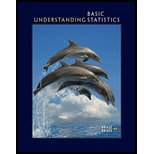Chapter 1.1, Problem 5P### Understanding Basic Statistics

8th Edition
Charles Henry Brase + 1 other
ISBN: 9781337558075

#### Solutions

Chapter
Section### Understanding Basic Statistics

8th Edition
Charles Henry Brase + 1 other
ISBN: 9781337558075
Textbook Problem

# Critical Thinking Numbers are often assigned to data that are categorical in nature.(a) Consider these number assignments for category items describing electronic ways of expressing personal opinions:1 = Twitter; 2 = e-mail; 3 = text message; 4 = Facebook; 5 = blogAre these numerical assignments at the ordinal data level or higher? Explain.(b) Consider these number assignments for category items describing usefulness of customer service:1 = not helpful; 2 = somewhat helpful; 3 = very helpful; 4 = extremely helpfulAre these numerical assignments at the ordinal data level? Explain. What about at the interval level or higher? Explain.

(a)

To determine

To explain: Whether the provided numerical assignments are at the ordinal data level or higher.

Explanation

The given values are 1—twitter, 2—email, 3—text message, 4—Facebook, and 5—blog and these values are nominal...

(b)

To determine

To explain: Whether provided numerical assignments are at the ordinal data level or higher.

### Still sussing out bartleby?

Check out a sample textbook solution.

See a sample solution

#### The Solution to Your Study Problems

Bartleby provides explanations to thousands of textbook problems written by our experts, many with advanced degrees!

Get Started

## Additional Math Solutions

#### Find more solutions based on key concepts